# Suppose that the matrix A A has the following eigenvalues and eigenvectors: (1 point) Suppose that... related homework questions

• #### It’s review question, I need this as soon as possible. Thank you 3) For thè diferential equation: (a) The point zo =-1 is an ordinary point. Compute the recursion formula for the coefficients of...It’s review question, I need this as soon as possible. Thank you 3) For thè diferential equation: (a) The point zo =-1 is an ordinary point. Compute the recursion formula for the coefficients of the power series solution centered at zo- -1 and use it to compute the first three nonzero terms of the power series when -1)-s and v(-1)-0....

• #### Find the matrix A that has the given eigenvalues and corresponding eigenvectors. Find the matrix A that has the given eigenvalues and corresponding eigenvectors. 2 A= Find the matrix A that has t...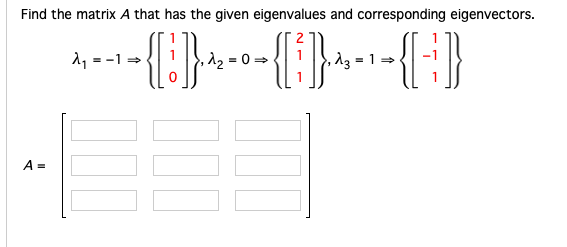Find the matrix A that has the given eigenvalues and corresponding eigenvectors. Find the matrix A that has the given eigenvalues and corresponding eigenvectors. 2 A= Find the matrix A that has the given eigenvalues and corresponding eigenvectors. 2 A=

• #### Dont copié formé thé book oh ya dont copié formé thé book cause you Oiil inde up being triste soi remembré not toi copié frome thé book oh ya

Dont copié formé thé book oh ya dont copié formé thé book cause you Oiil inde up being triste soi remembré not toi copié frome thé book oh ya!translation in english please!

• #### 4. Compute the eigenvalues and corresponding eigenvectors of the following matrix C 3 20 4. Compute the eigenvalues and corresponding eigenvectors of the following matrix C 3 20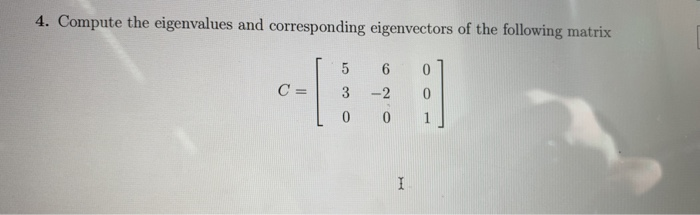4. Compute the eigenvalues and corresponding eigenvectors of the following matrix C 3 20 4. Compute the eigenvalues and corresponding eigenvectors of the following matrix C 3 20

• #### 8.2.35. Given an idempotent matrix, so that P = P2, find all its eigenvalues and eigenvectors. 8.2.35. Given an idempotent matrix, so that P = P2, find all its eigenvalues and eigenvectors.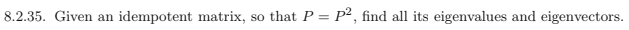8.2.35. Given an idempotent matrix, so that P = P2, find all its eigenvalues and eigenvectors. 8.2.35. Given an idempotent matrix, so that P = P2, find all its eigenvalues and eigenvectors.

• #### Problem 8. (15 points) Find eigenvalues and eigenvectors of the follwing matrix 3 -2 0 A= -1 3-2 0 -1 3 Problem 8. (15 points) Find eigenvalues and eigenvectors of the follwing matrix 3 -2 0...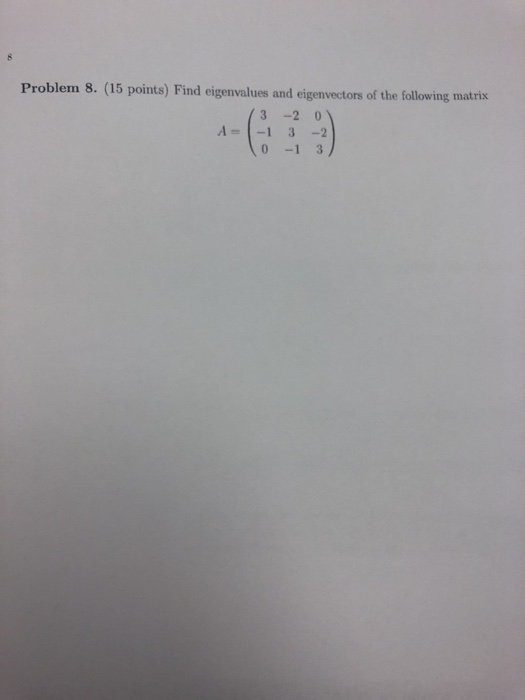Problem 8. (15 points) Find eigenvalues and eigenvectors of the follwing matrix 3 -2 0 A= -1 3-2 0 -1 3 Problem 8. (15 points) Find eigenvalues and eigenvectors of the follwing matrix 3 -2 0 A= -1 3-2 0 -1 3

• #### Find the eigenvalues and associated eigenvectors of the matrix Q2: Find the eigenvalues and associated eigenvectors...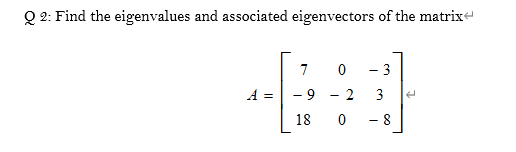Find the eigenvalues and associated eigenvectors of the matrix Q2: Find the eigenvalues and associated eigenvectors of the matrix 7 0 - 3 A = - 9 2 3 18 0 - 8

• #### What relationships are there between the rank/nullity and the eigenvalues/eigenvectors? How do the eigenvalues and eigenvectors...

What relationships are there between the rank/nullity and the eigenvalues/eigenvectors? How do the eigenvalues and eigenvectors of each matrix change under any arbitrary change of basis? What are some famous bases? What are they used for and why are they chosen for that particular application? (less than 500 words) What is the point of linear algebra (less than 200 words)...

• #### DSuppose \$39oo is deposited in a savings account that increases exponentially.Detamine thě APv if the acount...DSuppose \$39oo is deposited in a savings account that increases exponentially.Detamine thě APv if the acount increases to \$t020 in 4 years. Ass ume tne interest Vale remains Constant and no additional deposits or Withdrawals are made. (a.) Let pbe the APY. Note tnat if tme inital balaqe is yo, ne year later tne balane is %more. P- 3 (Tpe...

• #### Consider the 3 x 3 matrix A-1-ovvT where a R, 1 is the identity matrix and v the vector (a) Determine the eigenvalues and eigenvectors of A (b) Hence find a matrix which diagonalises A. (c) For which...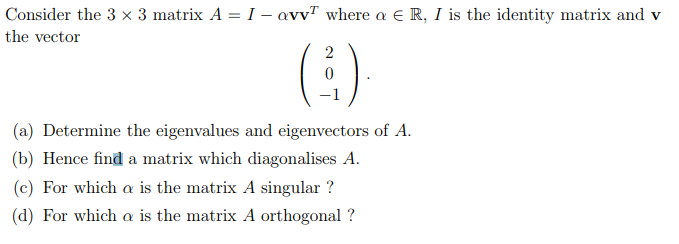Consider the 3 x 3 matrix A-1-ovvT where a R, 1 is the identity matrix and v the vector (a) Determine the eigenvalues and eigenvectors of A (b) Hence find a matrix which diagonalises A. (c) For which a is the matrix A singular? (d) For which α is the matrix A orthogonal ? Consider the 3 x 3 matrix...

• #### Suppose that the matrix A A has the following eigenvalues and eigenvectors: (1 point) Suppose that...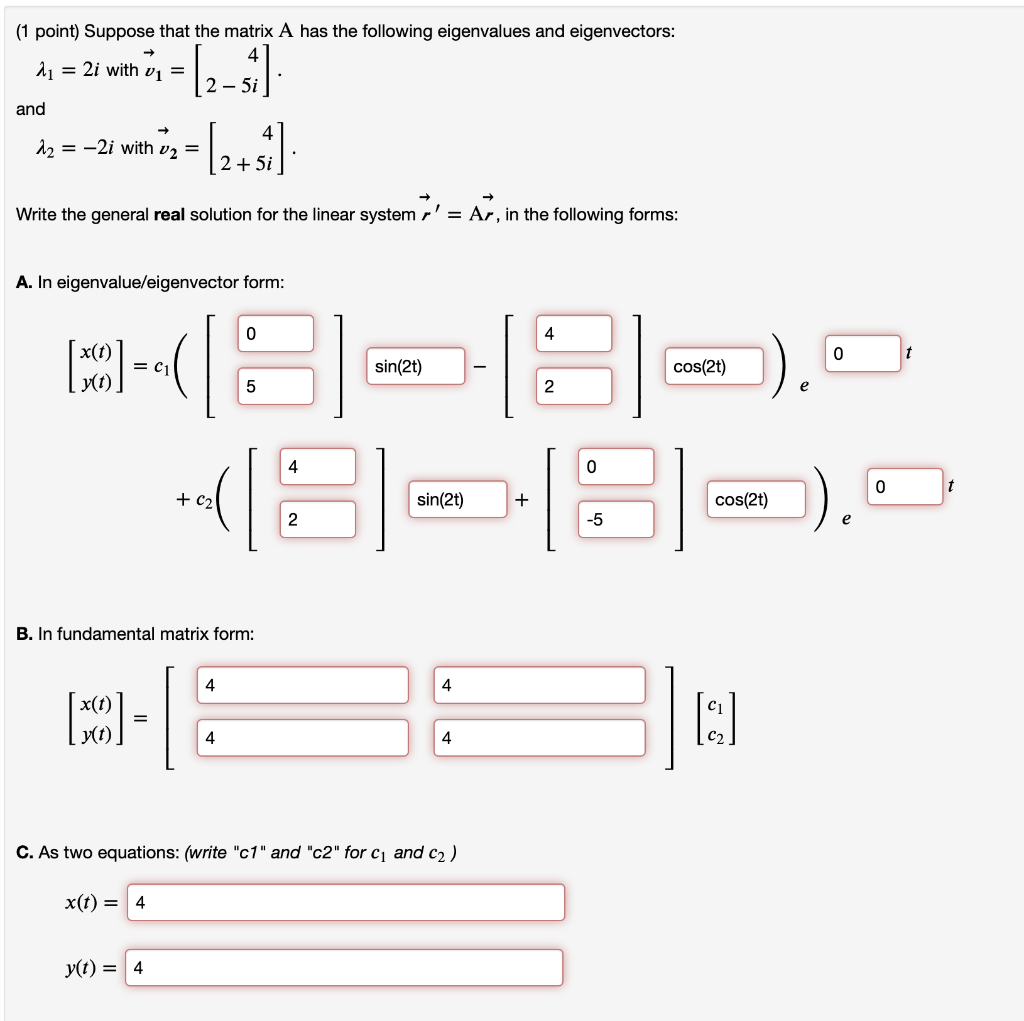Suppose that the matrix A A has the following eigenvalues and eigenvectors: (1 point) Suppose that the matrix A has the following eigenvalues and eigenvectors: 2 = 2i with v1 = 2 - 5i and - 12 = -2i with v2 = (2+1) 2 + 5i Write the general real solution for the linear system r' = Ar, in the...

• #### -2 2 4 2-5 (10 pts) The matrix C 12 3 has two distinct eigenvalues. AI < has multiplicity 2 and has multiplicity -2 2 4 2-5 (10 pts) The matrix C 12 3 has two distinct eigenvalues. AI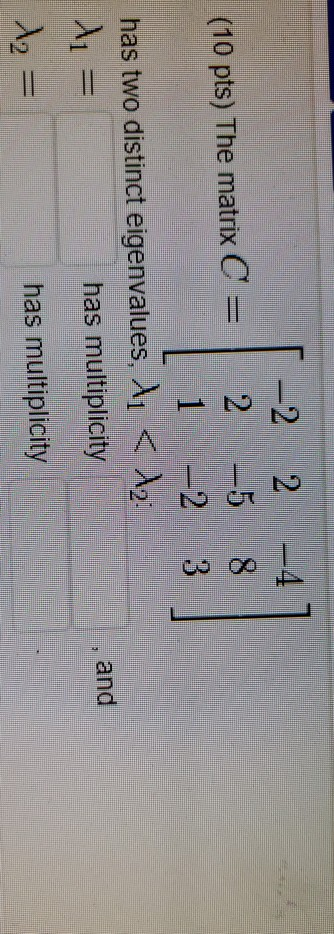-2 2 4 2-5 (10 pts) The matrix C 12 3 has two distinct eigenvalues. AI < has multiplicity 2 and has multiplicity -2 2 4 2-5 (10 pts) The matrix C 12 3 has two distinct eigenvalues. AI

• #### (1 point) Suppose that the matrix A has the following eigenvalues and eigenvectors 2-2i and -2+2i Write the solution to...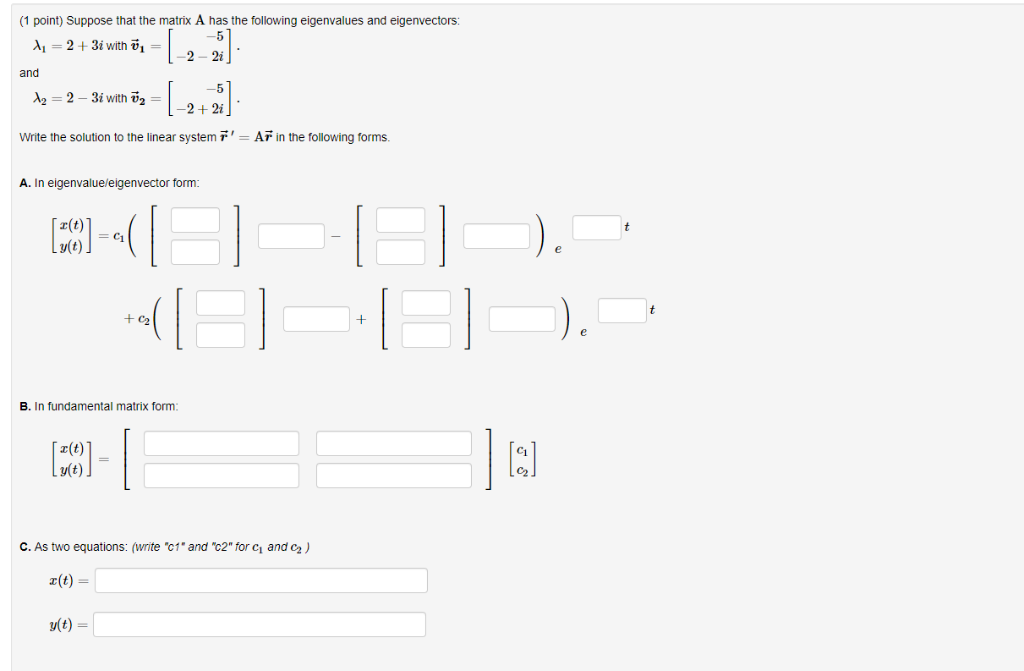(1 point) Suppose that the matrix A has the following eigenvalues and eigenvectors 2-2i and -2+2i Write the solution to the linear system AF in the following forms A. In eigenvalueleigenvector form r(t) B. In fundamental matrix form z(t) v(t) C. As two equations: (write "c1* and "c2" for ci and C2) a(t)- v(t)- (1 point) Suppose that the matrix...

• #### (1 point) Suppose that the matrix A has the following eigenvalues and eigenvectors: A1 = 4...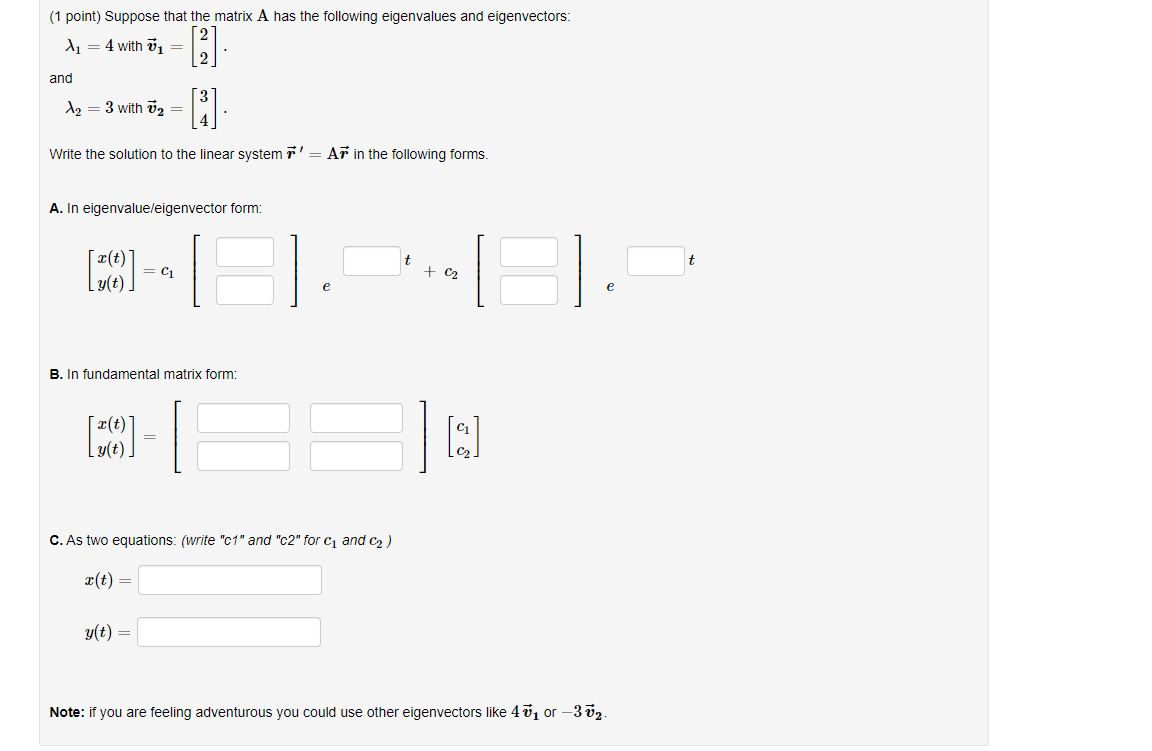(1 point) Suppose that the matrix A has the following eigenvalues and eigenvectors: A1 = 4 with = and  [i] Az = 3 with Ū2 = Write the solution to the linear system r' = Ar in the following forms. A. In eigenvalue/eigenvector form: t (10) -- + C2 e e B. In fundamental matrix form: (39) - g(t)....

• #### (1 point) Suppose that the matrix A has the following eigenvalues and eigenvectors: 4 = 2...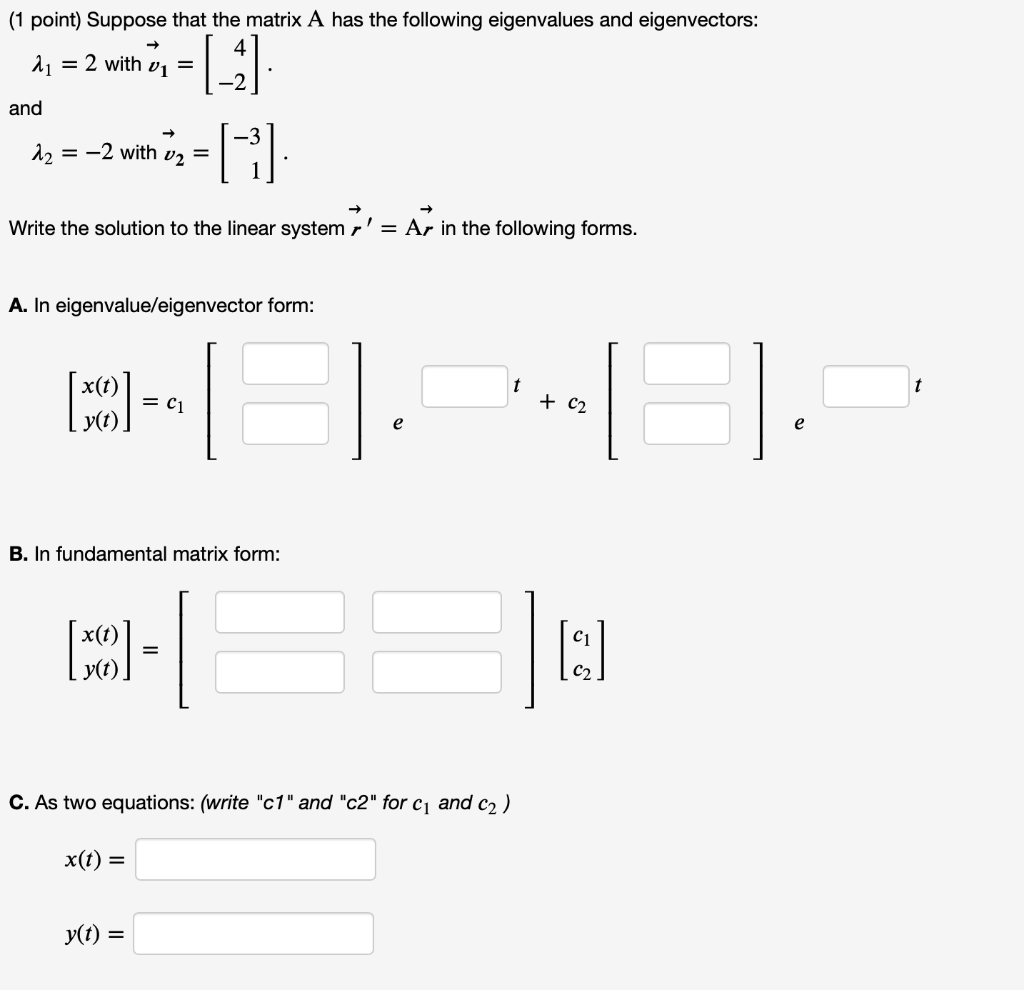(1 point) Suppose that the matrix A has the following eigenvalues and eigenvectors: 4 = 2 with vi = and |_ G 12 = -2 with v2 = Write the solution to the linear system r' = Ar in the following forms. A. In eigenvalue/eigenvector form: x(t) (50) = C1 + C2 e e B. In fundamental matrix form: (MCO)...

• #### Find the eigenvalues and normalized eigenvectors of the following matrices. Show whether the eigenvectors are orthogonal....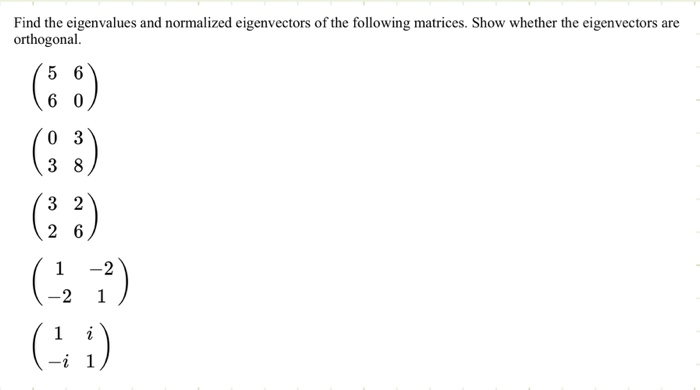Find the eigenvalues and normalized eigenvectors of the following matrices. Show whether the eigenvectors are orthogonal. (60) (23) (1, 1) (i)

• #### nd a. b such that the matrix has only two eigenvalues. nd a. b such that the matrix has only two eigenvalues.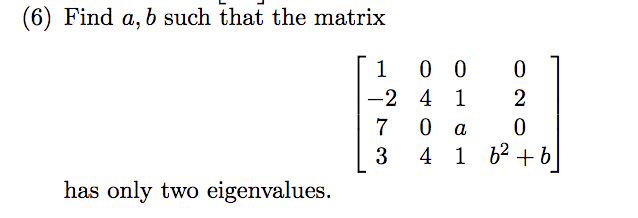nd a. b such that the matrix has only two eigenvalues. nd a. b such that the matrix has only two eigenvalues.

• #### Matrix Question!eigenvectors and eigenvalues for symmetric matrix?

Suppose A is a symmetric N X N matrix with eigenvectors vi, i = 1; 2; 3 ...N withcorresponding eigenvalues ?i, i = 1; 2; 3 ...N.Pick any two distinct eigenvalues (assuming such a pair exists). Let's call them ?1 and?2 and their corresponding eigenvectors v1 and v2.Write down the matrix equations that show that v1 and v2 are eigenvectors...

• #### 8. 20 pts.] Suppose that a 2 x2 matrix A has the following eigenvalues and eigenvectors:...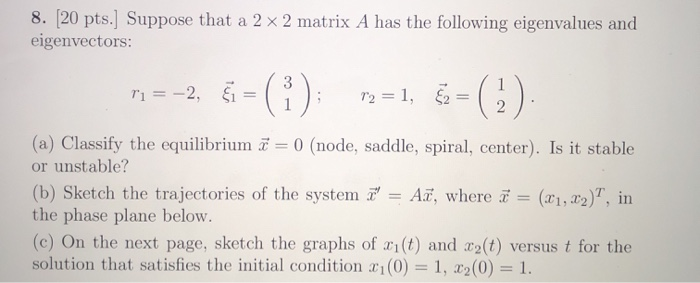8. 20 pts.] Suppose that a 2 x2 matrix A has the following eigenvalues and eigenvectors: () 12, 1 r2=1, 2 2 (a) Classify the equilibrium 0 (node, saddle, spiral, center). Is it stable or unstable? (b) Sketch the trajectories of the system A , where a the phase plane below. (c) On the next page, sketch the graphs of...

• #### Problem 3. For the following system, (a) compute the eigenvalues, (b) compute the associated eigenvectors, (c) if the eigenvalues are complex, determine if the origin is a spiral sink, a spiral s...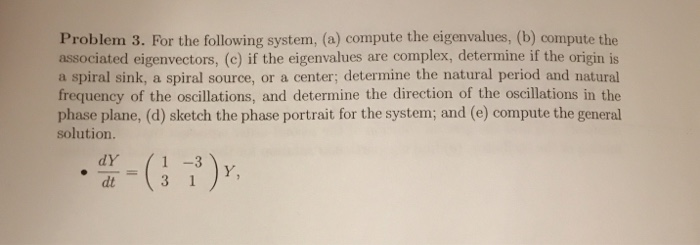Problem 3. For the following system, (a) compute the eigenvalues, (b) compute the associated eigenvectors, (c) if the eigenvalues are complex, determine if the origin is a spiral sink, a spiral source, or a center; determine the natural period and natural frequency of the oscillations, and determine the direction of the oscillations in the phase plane, (d) sketch the phase...

Free Homework App